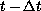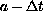Electron. J. Diff. Eqns., Vol. 1995(1995), No. 17, pp 1-14.

### Reflectionless Boundary Propagation Formulas for Partial Wave Solutions to the Wave Equation Jaime Navarro & Henry A. Warchall

Abstract:
We consider solutions to the wave equation in 3+1 spacetime dimensions whose data is compactly supported at some initial time. For points outside a ball containing the initial support, we develop an outgoing wave condition, and associated one-way propagation formula, for the partial waves in the spherical-harmonic decomposition of the solution. The propagation formula expresses the-th partial wave at time t and radius a in terms of order-l radial derivatives of the partial wave at timeand radius. The boundary propagation formula can be applied to any differential equation that is well-approximated by the wave equation outside a fixed ball.

Submitted May 20, 1994. Published November 28, 1995.
Math Subject Classification: 35L05, 35L15, 35C10, 35A35, 35A22.
Key Words: One-sided wave propagation, Wave equation, Reflectionless boundary conditions, Partial waves, Spherical-harmonic decomposition, Open-space boundary conditions.

Show me the PDF file (194 KB), TEX file, and other files for this article.Jaime Navarro Universidad Autonoma Metropolitana Unidad Azcapotzalco, Division de Ciencias Basicas Apdo. Postal 16-306, Mexico D.F. 02000, Mexico e-mail: jnfu@hp9000a1.uam.mxHenry A. Warchall Department of Mathematics, University of North Texas Denton TX 76203-5116, USA e-mail: hankw@unt.edu